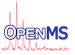OpenMS
Math

Math functions and classes. More...

Collaboration diagram for Math:

## Modules

Statistics functions
Various statistical functions.

Misc functions
Math functions.

## Classes

class  BilinearInterpolation< Key, Value >
Provides access to bilinearly interpolated values (and derivatives) from discrete data points. Values beyond the given range of data points are implicitly taken as zero. More...

class  LinearInterpolation< Key, Value >
Provides access to linearly interpolated values (and derivatives) from discrete data points. Values beyond the given range of data points are implicitly taken as zero. More...

class  BasicStatistics< RealT >
Calculates some basic statistical parameters of a distribution: sum, mean, variance, and provides the normal approximation. More...

Implements a fitter for the Gamma distribution. More...

class  GaussFitter
Implements a fitter for Gaussian functions. More...

class  GumbelDistributionFitter
Implements a fitter for the Gumbel distribution. More...

class  GumbelMaxLikelihoodFitter
Implements a fitter for the Gumbel distribution. More...

class  Histogram< ValueType, BinSizeType >
Representation of a histogram. More...

class  LinearRegression
This class offers functions to perform least-squares fits to a straight line model, $$Y(c,x) = c_0 + c_1 x$$. More...

class  LinearRegressionWithoutIntercept
This class offers functions to perform least-squares fits to a straight line model, $$Y(c,x) = c_0 + c_1 x$$. More...

class  PosteriorErrorProbabilityModel
Implements a mixture model of the inverse gumbel and the gauss distribution or a gaussian mixture. More...

class  ROCCurve
ROCCurves show the trade-off in sensitivity and specificity for binary classifiers using different cutoff values. More...

## Detailed Description

Math functions and classes.